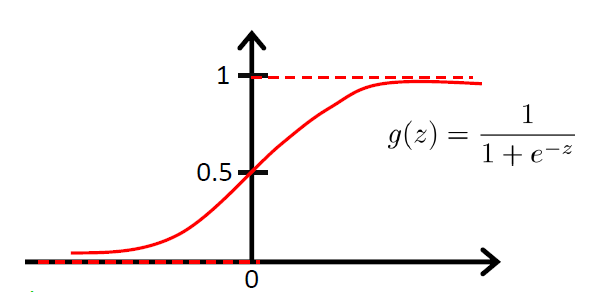# Halo

A magic place for coding

0%

## Intro

Logistic Regression，逻辑回归，实质上解决的是一个分类问题（我们之前说过，机器学习主要解决两类问题：回归和分类）。那为什么它又叫Regression呢？这是因为它用到了一个非线性函数——sigmoid函数，它给出的结果是一个概率值，而不是一个用于直接确定分类的离散值。下面我们就来仔细看看这个非常常用的分类算法。

## 原理

首先我们还是基于传统的线性回归（Linear Regression）去思考一个分类问题，假设我们的模型预测值是$h_\theta(x)$，这个值的范围是不确定的，我们很难去找到一个不错的阈值去划分两类（因为模型的输出会受数据分布的影响，0.5并不是一个很科学的阈值）。这个时候我们就需要将$h_\theta(x)$的值压缩到一个相对小的区间中，最理想的情况就是压缩到[0,1]中，这样输出的就可以是一个概率了。

幸运的是，我们能找到这样的函数——Sigmoid function（Logistic function）。它的曲线是这样的：从sigmoid的图像中我们可以看到一些我们想要的特性：

• 当$z\rightarrow\infty$时，$g(z)\rightarrow1$；当$z\rightarrow -\infty$时，$g(z)\rightarrow 0$

• $g(z)$的值被限制在了[0, 1]

• sigmoid是光滑的，他的导数也是很容易求得：
\begin{aligned} g^{‘}(x) &= \frac{d}{dz}\frac{1}{1+e^{-z}} \ &=\frac{1}{(1+e^{-z})^2}(e^{-z}) \ &= \frac{1}{(1+e^{-z})} \cdot(1 - \frac{1}{(1+e^{-z})}) \ &= g(z)(1-g(z)) \end{aligned}

于是模型的输出就变为：
$$h_\theta(x)=g(\theta^Tx)=\frac{1}{1+e^{-\theta^Tx}}$$
建立好模型后，我们的工作就是需要找到这个最佳的$\theta$。之前我们用的是LMS，但是这里使用LMS得到的并不是一个凸函数，我们需要换另一种方式——最大似然法。最大似然法的思路是：我已经知道了若干个测试结果，我需要找出一组参数$\theta$，使得它们能够很好地模拟出这些测试结果。将每一个模拟的概率乘起来，极大化这个概率，我们就能得到最好的$\theta$。下面我们就来表示这些概率。

首先我们假设：
\begin{aligned} &P(y=1|x;\theta)=h_\theta(x) \ &P(y=0|x;\theta)=1-h_\theta(x) \end{aligned}
从条件概率的角度看，这两条式子的意思是：对于给定的$x$和$\theta$，模型的输出就是$x$属于类别1的概率。这个可以看作一个二项分布问题，所以我们可以将两条式子合并到一起：
$$p(y|x;\theta)=(h_\theta(x))^y(1-h_\theta(x))^{1-y}$$
假设$m$个训练样本都是相互独立的，我们可以应用最大似然估计：
\begin{aligned} L(\theta) &= p(\hat{y}|X;\theta) \ &= \prod_{i=1}^mp(y^{(i)}|x^{(i)};\theta) \ &= \prod_{i=1}^m(h_\theta(x^{(i)}))^{y^{(i)}}(1-h_\theta(x^{(i)}))^{1-y^{(i)}} \end{aligned}
一般来说，我们都不会直接极大化$L(\theta)$，而是极大化它的对数：
\begin{aligned} l(\theta) &= logL(\theta) \ &= \sum_{i=1}^my^{(i)}logh(x^{(i)}) + (1-y^{(i)})log(1-h(x^{(i)})) \end{aligned}
极大化$l(\theta)$对我们来说就不是一件困难的事了，我们可以简单地使用GA（Gradient Ascend）。（特别注意，因为这里是要极大值，所以使用的Gradient Ascend）。推导过程如下：
\begin{aligned} \frac{\partial}{\partial\theta_j}l(\theta) &= (y\frac{1}{g(\theta^Tx)} -(1-y)\frac{1}{1-g(\theta^Tx)})\frac{\partial}{\partial\theta_j}g(\theta^Tx) \ &= (y\frac{1}{g(\theta^Tx)} -(1-y)\frac{1}{1-g(\theta^Tx)})g(\theta^Tx)(1-g(\theta^Tx))\frac{\partial}{\partial\theta_j}\theta^Tx \ &= (y(1-g(\theta^Tx))-(1-y)g(\theta^Tx))x_j \ &= (y-h_\theta(x))x_j \end{aligned}
因此，最后的更新规则为：
$$\theta_j := \theta_j+\alpha(y^{(i)} - h_\theta(x^{(i)}))x_j^{(i)}$$
有没有发现很眼熟？这个更新规则和LMS的更新规则是一样的！但是两者从本质上是不同的，这里的$h_\theta(x^{(i)})$是sigmoid函数。

至此，Logistic Regression的原理推导就完成了！大家最好能够自己手动推导一次。

## 实战

这里我用逻辑回归来预测疝气病马的存活问题。数据集来自UCI：传送门

### 数据预处理

之前的博客给过处理UCI数据的一些代码模板，需要特别注意，这次的数据存在许多缺漏，因此我们需要手动处理一下。

这里也简单地介绍一下如何处理缺失数据。处理缺失数据是特征工程一个很重要的部分，因为随便填补或删除会使得数据有可能加入了人为的噪声，所以我们需要有多种方法去填补。以下是几个典型的方法：

• 使用可用特征的均值（或众数）来填补缺失值；
• 使用特殊值来填补缺失值，如-1（相当于添加了一个新的类别）
• 直接忽略掉有缺失值的样本（对于缺失属性较多的样本可以这样做）
• 使用相似样本的均值填补缺失值
• 使用其他机器学习的方法预测缺失值（如使用随机森林来预测，相当于根据已知特征来预测缺失的特征）

对于这个样本，我的处理主要有两个：

• 使用0来填补缺失值，因为0对回归系数不会有影响，不会添加人为的bias。
• 如果某个样例的标签（label）缺失，则直接丢弃这个样例。（相当于不知道答案就不用做题）

### 构建Logistic回归分类器

定义sigmoid函数，使用梯度上升法求解。

### 随机梯度上升法

这里试用随机梯度上升法，每次梯度更新仅用到了一个样例。

### 构建输出部分

前面提到，Logistic Regression的输出是一个概率值，我们需要将这个概率值转化为一个类别，用0.5作为阈值是一个不错的选择。

### 测试

使用测试集来评估模型的效果。同时还可以对比两种不同的梯度上升法

测试结果：

• 随机梯度上升法，错误率约37%

• 常规的梯度上升法，错误率约29%

考虑到整个数据集的缺失率在30%左右，这个错误率也是可以接受的范围了。

## 使用Sklearn

使用Sklearn构建LogisticRegression模型非常简单，只需要定义几个变量就可以了。

这里简单解释一下模型的参数：

• solver：优化算法。选项为：
• liblinear：坐标下降法
• lbfgs：拟牛顿法，使用二阶导数来优化（海森矩阵）。
• newton-cg：牛顿法的一种。
• sag：随机平均梯度下降
• saga：SAG的加速版本

测试的结果正确率为73.529412%，似乎比我们自己写的要好一点点。

## 总结

Logistic Regression是机器学习中分类算法的基础，应用的范围非常广，实际效果也非常不错。一定要注意区分它和线性回归，它的数学原理推导部分是重点！至于实际应用，调用sklearn的模型就可以了。

Welcome to my other publishing channels